Name _______________________________ Date_____________

# Growing Animals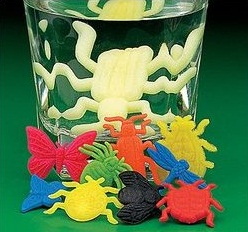Growing Objects can be purchased at toy stores.

Materials: Growing Objects (toys), ruler, water, warm water, tweezers

Question: How does water temperature affect the rate of growth of a sponge animal?

To answer this question, you will need to gather data as your "animal" grows and plot its size on a line graph.

## Experiment Design:

1.   Measure the length or weight of the objects at start time (Time 0)

2. Place one object in room temperature water, and the other object in warm water.

3. Measure the objects as they grow (approximately every 5 minutes)

Make a Prediction: Which one will grow faster, the one in warm water or the one in room temperature water?

## Data Table

 Object 1 Object 2 Time (min) Room Temperature Warm Water 0 (start) 5 10 15 20 25 30 35 40

## Graphing

Graph TWO LINES on the grid below. Place time on the X axis and length on the Y axis.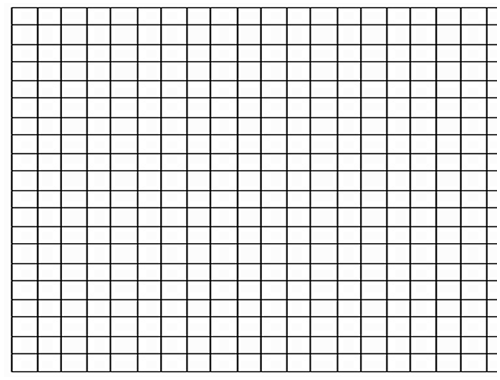### Analysis

1. Which type of water made the object grow faster? How do you know this for sure?

2. What metric unit was used in your measurement?

3. Examine this graph of worms as they grow. Assume that length is measured in centimeters.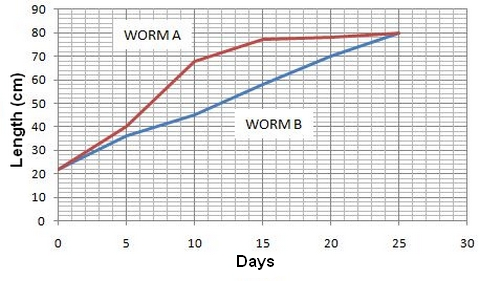A) Which worm had the fastest rate of growth? ______ Which worm had a constant rate of growth? ______

B) How big was worm A on Day 15 ? _____ How big was worm B on the same Day? _____

C) How big were both worms on day 25? _______

4. Growth rate can be measured by calculating he SLOPE of a line on a graph. To calcuate the slope, you need to pick two points on the line. Let's use WORM B to calculate a slope (m).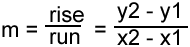What is the slope of WORM B's Line (show work above)? _____________

5. Once you have the slope (m) calculated, you can create an equation that can be used to PREDICT growth patterns.The equation for a line is

y = mx + b

m = the slope (you calculated in #4)
b = the point where the line crosses the Y axis, in our worm's case, b = 22

Write the full equation, plugging in the numbers for m and for b: __________________________________

6. Now that you have the EQUATION, you can use it to calculate how big your worm will be in on DAY 30.

7. The same number can also be estimated by extending the LINE out to Day 30 and using the graph. Show on the worm graph above where worm b's line would be on day 30 (assuming its growth remained constant).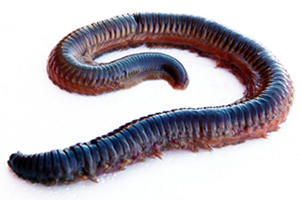8. How big will your worm be on Day 500 (assuming a constant rate of growth)?